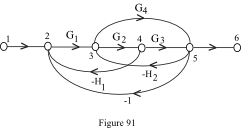### Signal flow graph

The graphical representation of the control system is known as signal flow graph. The system variables are represented with nodes. The path between the nodes is the branch. The path direction with the value represents the block of the system.

Example: Represent the block diagram with signal flow graph.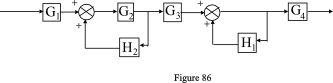Solution: Represent each summing point and takeoff point with nodes. Represent the branches with respective gains as shown in figure 87.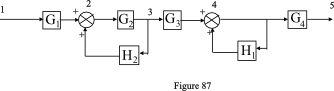Now draw the signal flowgraph using the nodes, branches and gains as shown in figure 88.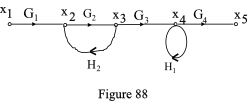## Terminology used in signal flow graph

1. Node: all the incoming and outgoing signals met a point is called a node. Let consider the figure 88, from this x1, x2,x3,x4 and x5 are the nodes. The equations will be,

x2=G1x1+H2x3
x4=G3x3+H1x4
x5=G4x4

1. Branch: a path with direction between nodes is called a branch.

2. Transmittance: the gain between the nodes or at nodes is called transmittance.

3. Input node/ source node: only outgoing branches from the node are input nodes. x1 is the input node from the figure 88.

4. Output node/ Sink node: only incoming branches at node are called output node. The x5 is the output node from the figure 88.

5. Chain node: both incoming and outgoing branches at a node are called chain nodes. The x2,x3 and x4 are called chain nodes.

6. Path: traveling a signal from one node to another node is called a path.

7. Forward path: the continuous unidirectional path from input to the output nodes is called forward path. From the figure 88, the x1,x2,x3,x4 and x5 is the forward path.

8. Closed loop: the loop which starts at node and reaches to the same node with unidirectional. From the figure 88, the x2,x3 and x2 is the closed loop.

9. Self loop: the loop which starts at node and reaches to the same node with unidirectional and having a single branch. From the figure 88, then x4 is a self loop.

10. Path gain: product of all branch gains in the path. From the figure 88, the x1,x2,x3,x4 and x5 is the forward path and the gain is G1G2G3G4G5..

11. Loop Gain:  product of branch gains in a closed loop. From the figure 88, the x2,x3 and x2 is the closed loop and the gain is G2H2.

Problem 13:

draw the signal flow graph for the block diagram as shown in figure 89.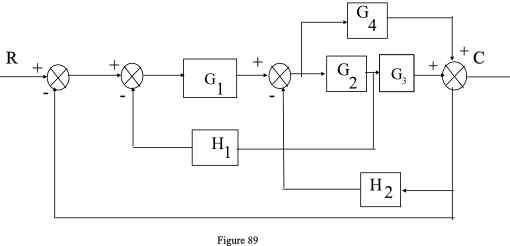Solution:

Identify the nodes in the block diagram as shown in figure 90.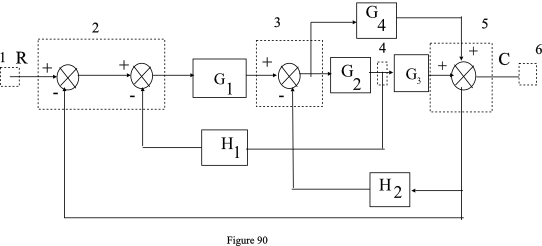After identifying the nodes, draw the signal flow graph as shown in the figure 91.